Publicité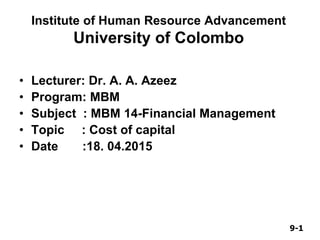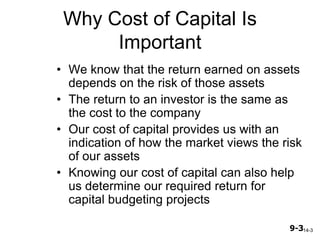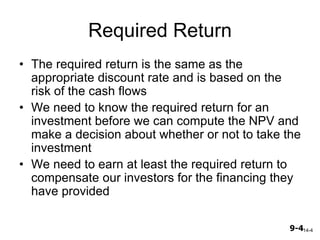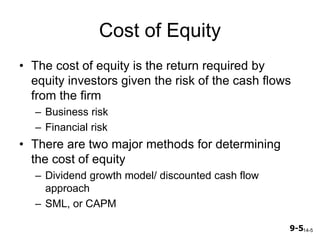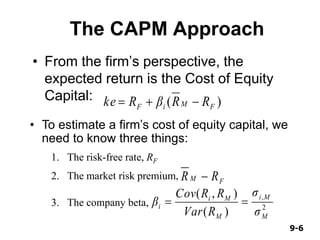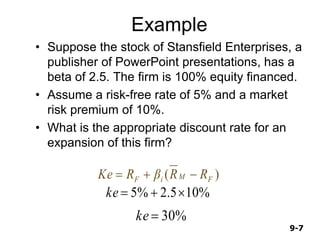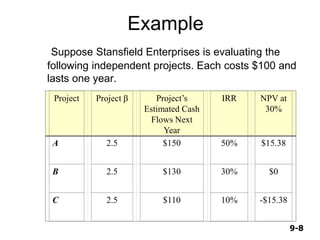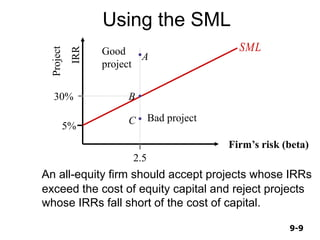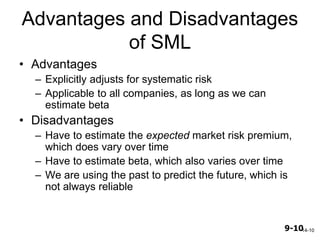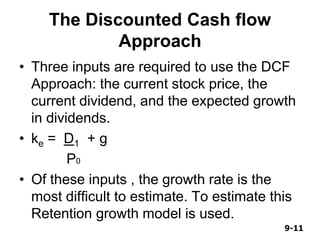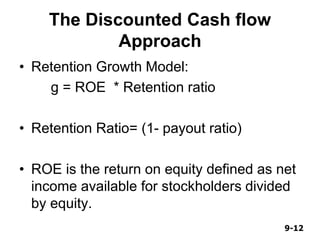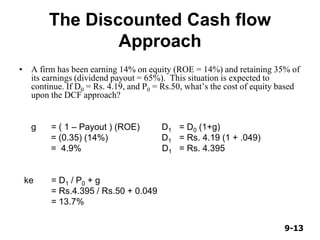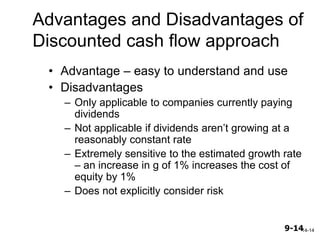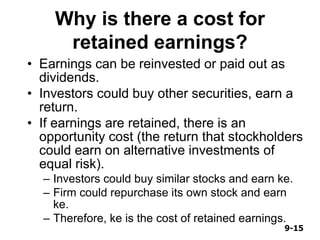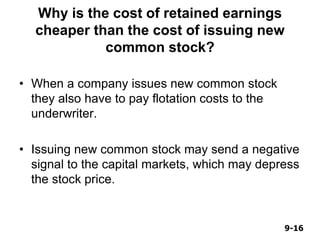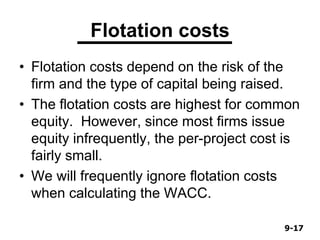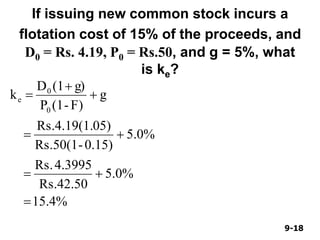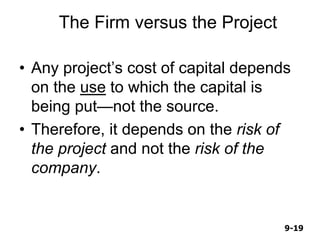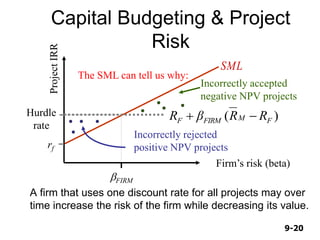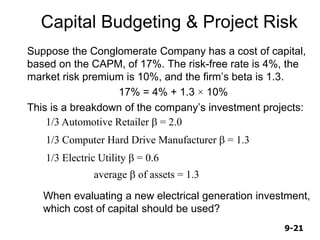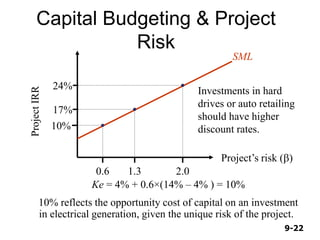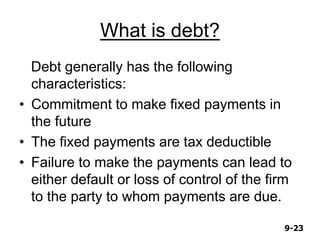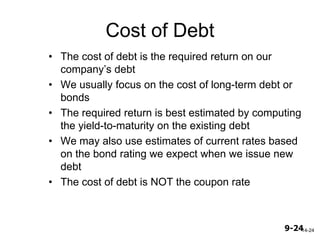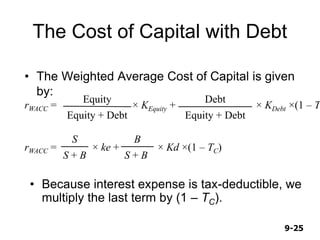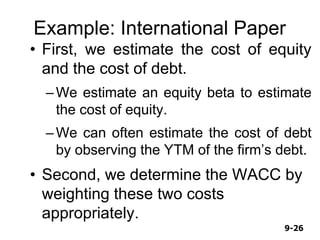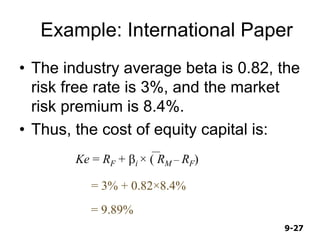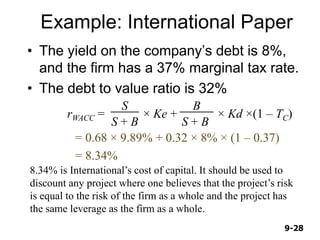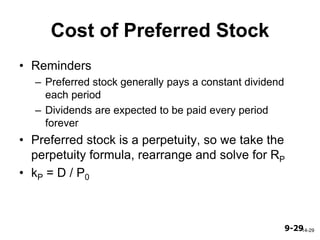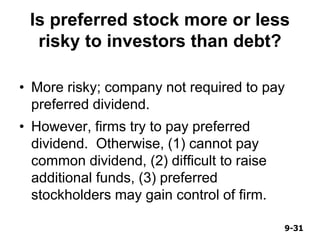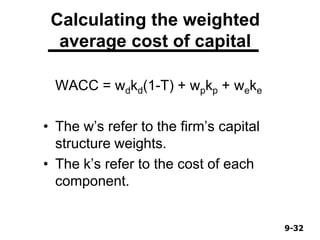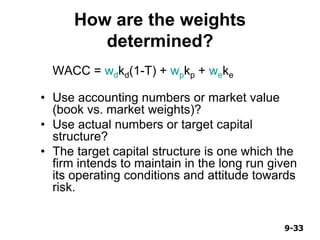1 sur 33
Publicité

### Cost of capital

1. 9-1 Institute of Human Resource Advancement University of Colombo • Lecturer: Dr. A. A. Azeez • Program: MBM • Subject : MBM 14-Financial Management • Topic : Cost of capital • Date :18. 04.2015
2. 9-2 Cost of Capital Firms raise funds from both equity investors and lenders to fund investments. Cost of Equity is the rate of return investors require on an equity investment in a firm. Cost of Capital is the required rate of return on the various types of financing. It is a weighted average of the individual required rates of return.
3. 9-3 Why Cost of Capital Is Important • We know that the return earned on assets depends on the risk of those assets • The return to an investor is the same as the cost to the company • Our cost of capital provides us with an indication of how the market views the risk of our assets • Knowing our cost of capital can also help us determine our required return for capital budgeting projects 14-3
4. 9-4 Required Return • The required return is the same as the appropriate discount rate and is based on the risk of the cash flows • We need to know the required return for an investment before we can compute the NPV and make a decision about whether or not to take the investment • We need to earn at least the required return to compensate our investors for the financing they have provided 14-4
5. 9-5 Cost of Equity • The cost of equity is the return required by equity investors given the risk of the cash flows from the firm – Business risk – Financial risk • There are two major methods for determining the cost of equity – Dividend growth model/ discounted cash flow approach – SML, or CAPM 14-5
6. 9-6 The CAPM Approach • From the firm’s perspective, the expected return is the Cost of Equity Capital: )( FMiF RRβRke  • To estimate a firm’s cost of equity capital, we need to know three things: 1. The risk-free rate, RF FM RR 2. The market risk premium, 2 , )( ),( M Mi M Mi i σ σ RVar RRCov β 3. The company beta,
7. 9-7 Example • Suppose the stock of Stansfield Enterprises, a publisher of PowerPoint presentations, has a beta of 2.5. The firm is 100% equity financed. • Assume a risk-free rate of 5% and a market risk premium of 10%. • What is the appropriate discount rate for an expansion of this firm? )( FMiF RRβRKe  %105.2%5 ke %30ke
8. 9-8 Example Suppose Stansfield Enterprises is evaluating the following independent projects. Each costs \$100 and lasts one year. Project Project b Project’s Estimated Cash Flows Next Year IRR NPV at 30% A 2.5 \$150 50% \$15.38 B 2.5 \$130 30% \$0 C 2.5 \$110 10% -\$15.38
9. 9-9 Using the SML An all-equity firm should accept projects whose IRRs exceed the cost of equity capital and reject projects whose IRRs fall short of the cost of capital. Project IRR Firm’s risk (beta) SML 5% Good project Bad project 30% 2.5 A B C
10. 9-10 Advantages and Disadvantages of SML • Advantages – Explicitly adjusts for systematic risk – Applicable to all companies, as long as we can estimate beta • Disadvantages – Have to estimate the expected market risk premium, which does vary over time – Have to estimate beta, which also varies over time – We are using the past to predict the future, which is not always reliable 14-10
11. 9-11 The Discounted Cash flow Approach • Three inputs are required to use the DCF Approach: the current stock price, the current dividend, and the expected growth in dividends. • ke = D1 + g P0 • Of these inputs , the growth rate is the most difficult to estimate. To estimate this Retention growth model is used.
12. 9-12 The Discounted Cash flow Approach • Retention Growth Model: g = ROE * Retention ratio • Retention Ratio= (1- payout ratio) • ROE is the return on equity defined as net income available for stockholders divided by equity.
13. 9-13 The Discounted Cash flow Approach • A firm has been earning 14% on equity (ROE = 14%) and retaining 35% of its earnings (dividend payout = 65%). This situation is expected to continue. If D0 = Rs. 4.19, and P0 = Rs.50, what’s the cost of equity based upon the DCF approach? g = ( 1 – Payout ) (ROE) D1 = D0 (1+g) = (0.35) (14%) D1 = Rs. 4.19 (1 + .049) = 4.9% D1 = Rs. 4.395 ke = D1 / P0 + g = Rs.4.395 / Rs.50 + 0.049 = 13.7%
14. 9-14 Advantages and Disadvantages of Discounted cash flow approach • Advantage – easy to understand and use • Disadvantages – Only applicable to companies currently paying dividends – Not applicable if dividends aren’t growing at a reasonably constant rate – Extremely sensitive to the estimated growth rate – an increase in g of 1% increases the cost of equity by 1% – Does not explicitly consider risk 14-14
15. 9-15 Why is there a cost for retained earnings? • Earnings can be reinvested or paid out as dividends. • Investors could buy other securities, earn a return. • If earnings are retained, there is an opportunity cost (the return that stockholders could earn on alternative investments of equal risk). – Investors could buy similar stocks and earn ke. – Firm could repurchase its own stock and earn ke. – Therefore, ke is the cost of retained earnings.
16. 9-16 Why is the cost of retained earnings cheaper than the cost of issuing new common stock? • When a company issues new common stock they also have to pay flotation costs to the underwriter. • Issuing new common stock may send a negative signal to the capital markets, which may depress the stock price.
17. 9-17 Flotation costs • Flotation costs depend on the risk of the firm and the type of capital being raised. • The flotation costs are highest for common equity. However, since most firms issue equity infrequently, the per-project cost is fairly small. • We will frequently ignore flotation costs when calculating the WACC.
18. 9-18 If issuing new common stock incurs a flotation cost of 15% of the proceeds, and D0 = Rs. 4.19, P0 = Rs.50, and g = 5%, what is ke? 15.4% 5.0% Rs.42.50 4.3995Rs. 5.0% 0.15)-Rs.50(1 05)Rs.4.19(1. g F)-(1P g)(1D k 0 0 e      
19. 9-19 The Firm versus the Project • Any project’s cost of capital depends on the use to which the capital is being put—not the source. • Therefore, it depends on the risk of the project and not the risk of the company.
20. 9-20 Capital Budgeting & Project Risk A firm that uses one discount rate for all projects may over time increase the risk of the firm while decreasing its value. ProjectIRR Firm’s risk (beta) SML rf bFIRM Incorrectly rejected positive NPV projects Incorrectly accepted negative NPV projects Hurdle rate )( FMFIRMF RRβR  The SML can tell us why:
21. 9-21 Suppose the Conglomerate Company has a cost of capital, based on the CAPM, of 17%. The risk-free rate is 4%, the market risk premium is 10%, and the firm’s beta is 1.3. 17% = 4% + 1.3 × 10% This is a breakdown of the company’s investment projects: 1/3 Automotive Retailer b = 2.0 1/3 Computer Hard Drive Manufacturer b = 1.3 1/3 Electric Utility b = 0.6 average b of assets = 1.3 When evaluating a new electrical generation investment, which cost of capital should be used? Capital Budgeting & Project Risk
22. 9-22 Capital Budgeting & Project Risk ProjectIRR Project’s risk (b) 17% 1.3 2.00.6 Ke = 4% + 0.6×(14% – 4% ) = 10% 10% reflects the opportunity cost of capital on an investment in electrical generation, given the unique risk of the project. 10% 24% Investments in hard drives or auto retailing should have higher discount rates. SML
23. 9-23 What is debt? Debt generally has the following characteristics: • Commitment to make fixed payments in the future • The fixed payments are tax deductible • Failure to make the payments can lead to either default or loss of control of the firm to the party to whom payments are due.
24. 9-24 Cost of Debt • The cost of debt is the required return on our company’s debt • We usually focus on the cost of long-term debt or bonds • The required return is best estimated by computing the yield-to-maturity on the existing debt • We may also use estimates of current rates based on the bond rating we expect when we issue new debt • The cost of debt is NOT the coupon rate 14-24
25. 9-25 The Cost of Capital with Debt • The Weighted Average Cost of Capital is given by: • Because interest expense is tax-deductible, we multiply the last term by (1 – TC). rWACC = Equity + Debt Equity × KEquity + Equity + Debt Debt × KDebt ×(1 – T rWACC = S + B S × ke + S + B B × Kd ×(1 – TC)
26. 9-26 Example: International Paper • First, we estimate the cost of equity and the cost of debt. –We estimate an equity beta to estimate the cost of equity. –We can often estimate the cost of debt by observing the YTM of the firm’s debt. • Second, we determine the WACC by weighting these two costs appropriately.
27. 9-27 Example: International Paper • The industry average beta is 0.82, the risk free rate is 3%, and the market risk premium is 8.4%. • Thus, the cost of equity capital is: Ke = RF + bi × ( RM – RF) = 3% + 0.82×8.4% = 9.89%
28. 9-28 Example: International Paper • The yield on the company’s debt is 8%, and the firm has a 37% marginal tax rate. • The debt to value ratio is 32% 8.34% is International’s cost of capital. It should be used to discount any project where one believes that the project’s risk is equal to the risk of the firm as a whole and the project has the same leverage as the firm as a whole. = 0.68 × 9.89% + 0.32 × 8% × (1 – 0.37) = 8.34% rWACC = S + B S × Ke + S + B B × Kd ×(1 – TC)
29. 9-29 Cost of Preferred Stock • Reminders – Preferred stock generally pays a constant dividend each period – Dividends are expected to be paid every period forever • Preferred stock is a perpetuity, so we take the perpetuity formula, rearrange and solve for RP • kP = D / P0 14-29
30. 9-30 Example: Cost of Preferred Stock • Your company has preferred stock that has an annual dividend of \$3. If the current price is \$25, what is the cost of preferred stock? • KP = 3 / 25 = 12% 14-30
31. 9-31 Is preferred stock more or less risky to investors than debt? • More risky; company not required to pay preferred dividend. • However, firms try to pay preferred dividend. Otherwise, (1) cannot pay common dividend, (2) difficult to raise additional funds, (3) preferred stockholders may gain control of firm.
32. 9-32 Calculating the weighted average cost of capital WACC = wdkd(1-T) + wpkp + weke • The w’s refer to the firm’s capital structure weights. • The k’s refer to the cost of each component.
33. 9-33 How are the weights determined? WACC = wdkd(1-T) + wpkp + weke • Use accounting numbers or market value (book vs. market weights)? • Use actual numbers or target capital structure? • The target capital structure is one which the firm intends to maintain in the long run given its operating conditions and attitude towards risk.
Publicité